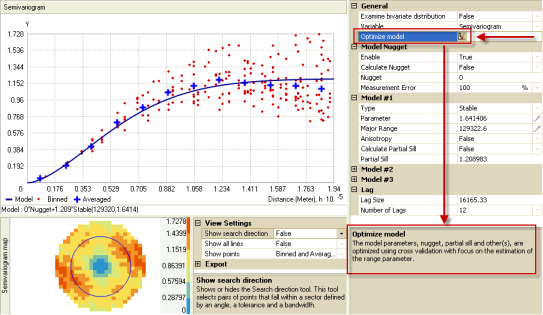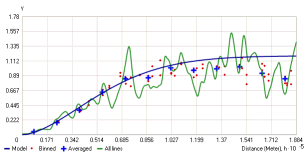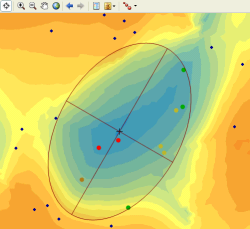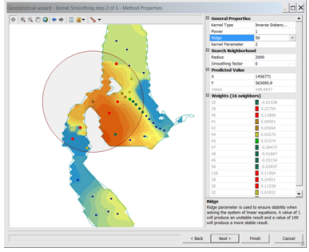What's new in ArcGIS Geostatistical Analyst 10
What's new in ArcGIS Geostatistical Analyst 10

The ArcGIS Geostatistical Analyst extension provides a broad range of powerful spatial modeling and analysis capabilities. With version 10, Geostatistical Analyst provides improvements in performance and new tools.

## New geoprocessing tools

There are 11 new geoprocessing tools for Geostatistical Analyst in ArcGIS 10.

Of these tools, 4 are new functionality, and the remaining 7 were previously only available through the Geostatistical Wizard or from the Geostatistical Analyst toolbar.

### Diffusion Interpolation With Barriers

Diffusion Interpolation With Barriers uses a kernel that is based on the heat equation and allows you to use a combination of raster and feature datasets to act as barriers.

### Kernel Interpolation With Barriers

Kernel Interpolation With Barriers is a moving window predictor that uses the shortest distance between points. The illustration below shows the paths from the data locations (black circles) to the location where a prediction (red square) is required.

### Global Polynomial Interpolation

Global Polynomial Interpolation is like taking a piece of paper and fitting it between the raised points (raised to the height of value). It is also often referred to as Trend Surface Analysis.

### Local Polynomial Interpolation

Local Polynomial Interpolation fits many polynomials, each within specified overlapping neighborhoods. New functionality for this tool includes the ability to create a Prediction Standard Error surface and also the inclusion of optimization and diagnostic routines.

### IDW

IDW interpolation explicitly implements the assumption that things that are close to one another are more alike than those that are farther apart. It weights the points closer to the prediction location greater than those farther away—hence, the name inverse distance weighted.

Radial Basis Functions methods are a series of exact interpolation techniques; that is, the surface must go through each measured sample value.

### Create Spatially Balanced Points

Create Spatially Balanced Points generates a set of sample points based on a priori inclusion probabilities. The resulting sample design is spatially balanced, meaning that the spatial independence between samples is maximized, making the design more efficient than sampling the study area at random.

### Densify Sampling Network

Densify Sampling Network is based on a predefined geostatistical kriging layer. It uses, inter alia, the Standard Error of Prediction surface, to determine where new locations are required or which can be removed.

### Extract Values To Table

Extract Values to Table extracts the cell values from a set of rasters, based on a point or polygon feature class, to a table. If a point feature class is used, the output table has a record for each point and each raster that has data. Polygonal data is treated as point data; the cell center of the input rasters determines the number of points and is used to decide whether the cell is contained within the polygon or not. This tool can be used to further analyze the results from the Gaussian Geostatistical Simulations tool.

### Cross Validation

Cross Validation uses the idea of removing one data location and predicting the associated data using the data at the rest of the locations, then repeating this for the remaining locations. In this way, you can compare the predicted value to the observed value and obtain useful information about some of your decisions on the model.

### Subset Features

Subset Features employs one of the most rigorous ways to assess the quality of an output surface by comparing the predicted values with those measured in the field. One solution is to divide the original dataset into two parts. One part can be used to model the spatial structure and produce a surface, and the other part can be used to compare and validate the output surface.

## Improvements to the Wizard

The Geostatistical Wizard is a dynamic set of pages that are designed to guide you through the process of constructing and evaluating the performance of an interpolation model.

### Resizable windows

• The overall window can be resized.
• Individual panels can be resized.

### New dialog box layout and functionality

• Many of the model parameters can be optimized using cross validation.
• Parameter help is available on the dialog box and from the compiled help when additional help is required.Geostatistical Analyst Wizard

### Visualization of the semivariogram

• Views include the original binned values, one averaged value per bin, and a smoothed line that shows the data correlation in different directions.### Surface Preview

• The surface preview is changed so that the dataset preview and the surface preview can be turned on and off.### New Interpolation Methods

• Diffusion Interpolation With Barriers and Kernel Interpolation With Barriers are two new interpolation methods available in the Wizard and also as individual geoprocessing tools. The illustration below is of silt depth in a lake, and the boundary of the lake constrains the searching neighborhood.Example of interpolation methods in the wizard

### Local Polynomial Interpolation is enhanced to include inter alia

• Prediction standard errors indicate the uncertainty associated with the value predicted for each location.
• Condition numbers shows variation in the numerical model stability and provides additional information on the prediction uncertainty, since the prediction standard error surface is created assuming that the model is correct, so that there is no reason for the model instability.
• Optimize Model modifies the Bandwidth, Spatial Condition Number, and Neighborhood values; then the cross validation statistics are used to optimize the model.

## Further enhancements

### A new Conditioning measurement error field

A new Conditioning measurement error field is added to the Gaussian Geostatistical Simulations tool. It is used when a constant measurement error for all input data can be specified in the input semivariogram model. However, if the measurement error values are not the same at each sampling location, they can be specified using this field.

### Large dataset handling

• Some of the interpolation methods can now manage very large input datasets.
• IDW with roughly 2 billion input points (contained in more than 400,000 multipoints) produced an output raster of 250 by 250 columns and rows in about 20 hours.

### New and updated help

For example, A quick tour of Geostatistical Analyst has been added.

### New tutorials

Introduction to the ArcGIS Geostatistical Analyst Tutorial has been updated.

Source: http://help.arcgis.com